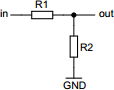# Resistive voltage divider

## Calculates resistors of the voltage divider network automatically. Assumes the required output current to be negligible.

 Example 1: Must calculate the resistors for a 5V to 1.25V voltage divider using 1% resistor series View example
 Input voltage volts Output voltage volts Resistor series E6 (20%)E12 (10%)E24 (5%)E48 (2%)E96 (1%)E192 (>1%) Min resistance ohms Max resistance ohmsYou may use one of the following SI prefix after a value: p=pico, n=nano, u=micro, m=milli, k=kilo, M=mega, G=giga

Fill in all the fields. The result will be a list of best matches found.
• Input voltage - Known input voltage
• Output voltage - Required output voltage with the given input voltage
• Resistor series - Resistor E-series of where to choose resistors from
• Min resistance - Minimum value of the resistors (can be 1ohm-100Mohm)
• Max resistance - Maximum value of the resistors (can be 1ohm-100Mohm)